1800-1023-196

+91-120-4616500

# Balancing of Oxidation-Reduction Reactions

## Balancing of Oxidation-Reduction Reactions

Though there are a number of methods for balancing oxidation – reduction reactions, two methods are very important. These are,

(1) Oxidation number method

(2) Ion – electron method

(1) Oxidation number method: The method for balancing redox reactions by oxidation number change method was developed by Johnson. In a balanced redox reaction, total increase in oxidation number must be equal to the total decrease in oxidation number. This equivalence provides the basis for balancing redox reactions. This method is applicable to both molecular and ionic equations. The general procedure involves the following steps,

(i) Write the skeleton equation (if not given, frame it) representing the chemical change.

(ii) Assign oxidation numbers to the atoms in the equation and find out which atoms are undergoing oxidation and reduction. Write separate equations for the atoms undergoing oxidation and reduction.

(iii) Find the change in oxidation number in each equation. Make the change equal in both the equations by multiplying with suitable integers. Add both the equations.

(iv) Complete the balancing by inspection. First balance those substances which have undergone change in oxidation number and then other atoms except hydrogen and oxygen. Finally balance hydrogen and oxygen by putting H2O molecules wherever needed.

The final balanced equation should be checked to ensure that there are as many atoms of each element on the right as there are on the left.

(v) In ionic equations the net charges on both sides of the equation must be exactly the same. Use H+ ion/ions in acidic reactions and OH ion/ions in basic reactions to balance the charge and number of hydrogen and oxygen atoms.

The following example illustrate the above rules,

Step : I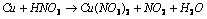(Skeleton equation)

Step: II Writing the oxidation number of all the atoms.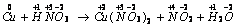Step: III Change in oxidation number has occurred in copper and nitrogen.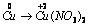......(i)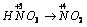......(ii)

Increase in oxidation number of copper = 2 units per molecule Cu

Decrease in oxidation number of nitrogen = 1 unit per molecule HNO3

Step: IV To make increase and decrease equal, equation (ii) is multiplied by 2.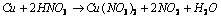Step: V Balancing nitrate ions, hydrogen and oxygen, the following equation is obtained.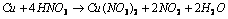This is the balanced equation.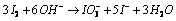## NEET & AIIMS Exam Sample Papers

 AIIMS SAMPLE PAPERS View More NEET SAMPLE PAPERS View More